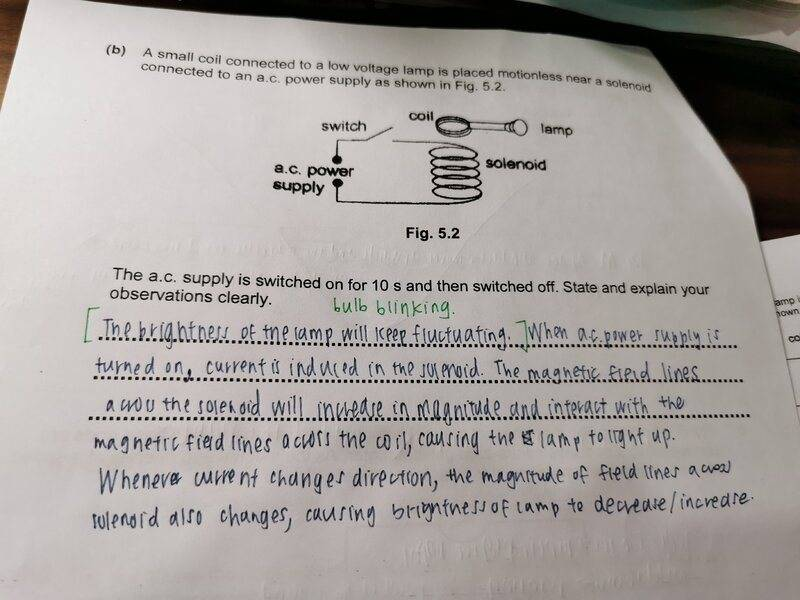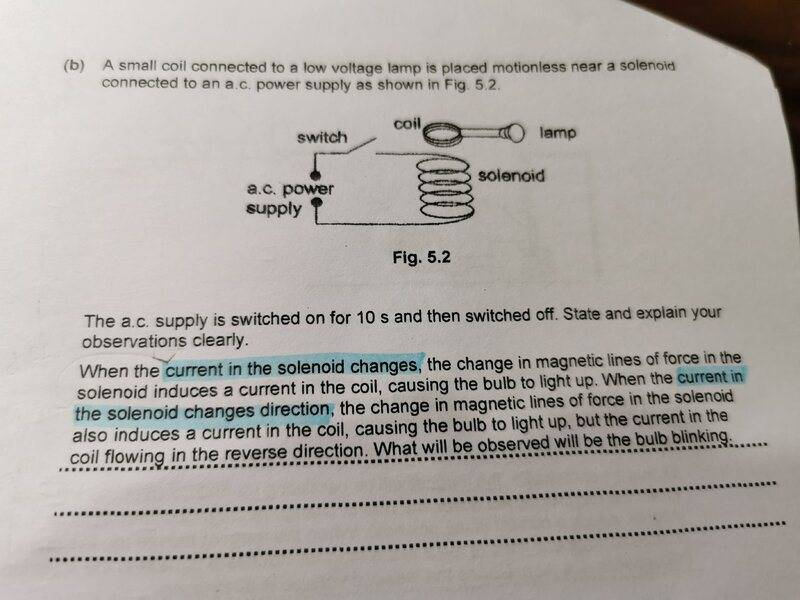# Question about how light bulb brightness varies when an AC supply is used

ellieee
Homework Statement:
in pic
just wanted to check if my answer is acceptable
Relevant Equations:
nilwillem2
Homework Statement:: State and explain your observations clearly.
just wanted to check if my answer is acceptable
Relevant Equations:: nil
Did you actually observe that the bulb was flashing?
The 2 coils of wire are really just a bad transformer, and if you input 50 or 60 Hz AC, you will get 50 or 60 Hz AC out. An incandescent lamp will actually flash at twice the frequency, so 100 or 120 Hz. (if the ac voltage is proportional to sin( at), the energy produced in the lamp is sin2(at). and this has double the frequency).
I don't think you can see that with the naked eye. You can probably with a phone camera, if you look at individual pictures.

•Delta2
Homework Helper
2022 Award
the energy produced in the lamp is sin2(at). and this has double the frequency).
Also there is a thermal time constant for any filament that is longer usually much longer than the 10 ms time of the AC. The light will not "blink". It will flicker slightly at the 100 (or 120) Hz.

•rsk
Your thread title says "how light bulb brightness varies when an AC supply is used" and yet I don't see anything in the question that suggests there will be any visible variation other than on and off- Is that your interpretation of the question or was it explicitly stated in the first part of the question ?

The answer required here will depend on the level of the course being followed, but this could easily be taken from an A-level paper. In that case, the answer would include the following points:

When the switch is closed an alternating current flows in the solenoid
This produces an alternating (or changing) magnetic field
Therefore there is a changing flux linkage in the coil
Therefore an alternating emf is induced in the coil
Since there is a closed circuit, the induced emf produces a current and the bulb lights up.

then of course, when the ac supply is switched off, all of this stops.

Homework Helper
2022 Award
I wanted to point out that the light will appear "on" and not "blinking". In fact there are two reasons: the thermal issues I mentioned and the flicker fusion rate of your eye.
Therefore there is a changing flux linkage in the coil
I believe this is incorrect use of the term "flux linkage". It describes the flux geometry. There will be a changing flux thru the secondary coil. The flux linkage changes if you move the coil. Not a huge deal

•rsk
Homework Helper
Gold Member
•rsk
rsk
I wanted to point out that the light will appear "on" and not "blinking". In fact there are two reasons: the thermal issues I mentioned and the flicker fusion rate of your eye.

I believe this is incorrect use of the term "flux linkage". It describes the flux geometry. There will be a changing flux thru the secondary coil. The flux linkage changes if you move the coil. Not a huge deal
Noted, thanks :)

•hutchphd
Homework Helper
Gold Member
An incandescent lamp will actually flash at twice the frequency, so 100 or 120 Hz. (if the ac voltage is proportional to sin( at), the energy produced in the lamp is sin2(at). and this has double the frequency).
Why are you saying that the bulb will flash at the frequency of the supplied energy? (which is indeed double the frequency of the supplied voltage or current). The way I know it the bulb blinks at the frequency of the supplied current, however it will not blink due to thermal considerations as @hutchphd notes.

rsk
Watch this demo.
That's fab - so simple but so effective.

willem2
Why are you saying that the bulb will flash at the frequency of the supplied energy? (which is indeed double the frequency of the supplied voltage or current). The way I know it the bulb blinks at the frequency of the supplied current, however it will not blink due to thermal considerations as @hutchphd notes.
Because the energy is what is heating up the bulb making it glow. Also the direction of the current doesn't matter for the amount of light produced.
If you used a single LED with a resistor, The amount of light would be proportional to the current, and you would only get half the frequency of incandescent light bulbs.

Homework Helper
Gold Member
Because the energy is what is heating up the bulb making it glow. Also the direction of the current doesn't matter for the amount of light produced.
If you used a single LED with a resistor, The amount of light would be proportional to the current, and you would only get half the frequency of incandescent light bulbs.
What's the difference between a LED and a bulb?

Homework Helper
2022 Award
Because the energy is what is heating up the bulb making it glow. Also the direction of the current doesn't matter for the amount of light produced.
If you used a single LED with a resistor, The amount of light would be proportional to the current, and you would only get half the frequency of incandescent light bulbs.
But the incandescent bulb light output is mostly DC with a small (<10% typically) 120 Hz ripple on top because of the thermal response time. An LED response at this time scale is instant (I have driven them at 70 MHz successfully) and they will be off for half the cycle.

••